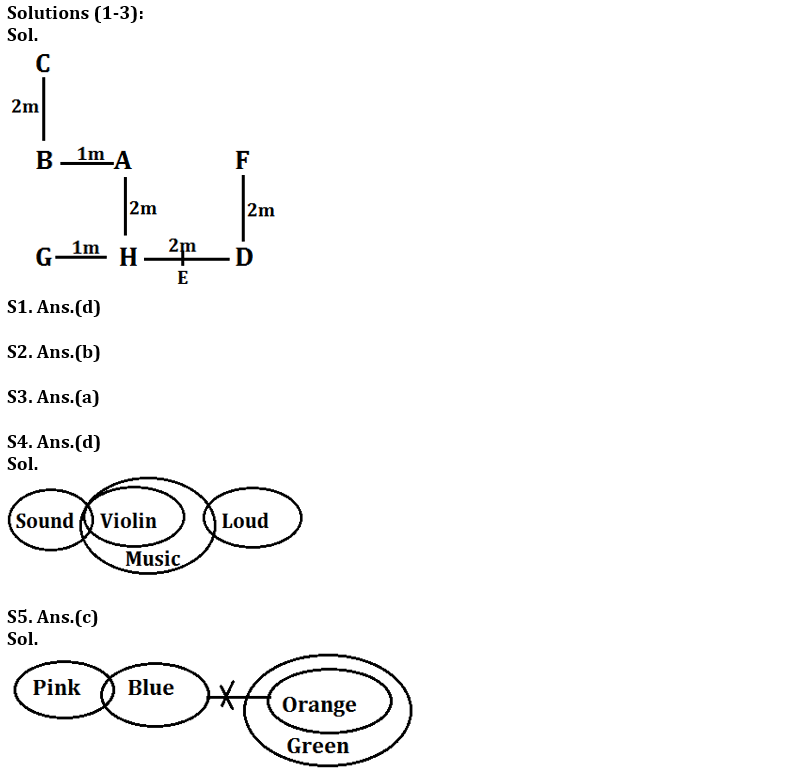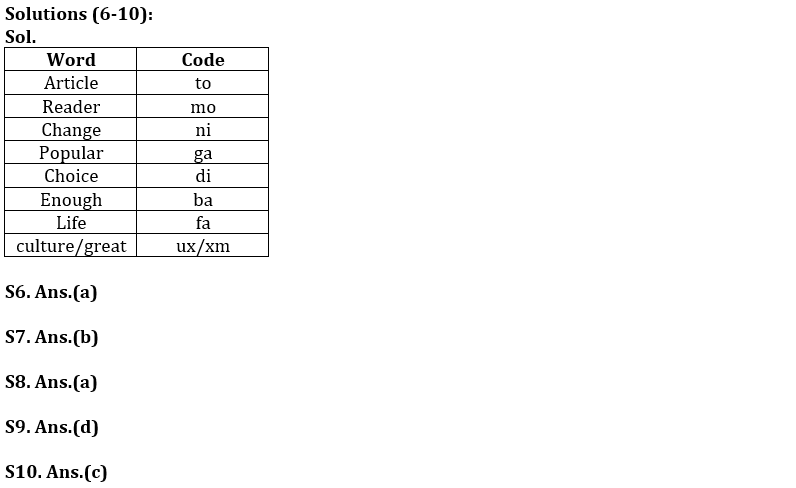Latest Banking jobs   »   Reasoning Ability Quiz For Bank Foundation...

# Reasoning Ability Quiz For Bank Foundation 2023 – 07th May

Directions (1-3): Study the information given below carefully and answer the questions that follow:
Point C is 2 m north of Point B. Point A is 1 m east of B and Point H is 2 m south of A. Point G is 1 m west of point H while Point D is 3 m east of point G and Point F is 2 m north of point D. Point E is just in middle of point H and point D.

Q1. If Point K is 2m north of point F, then what is the shortest distance between point G to point K?
(a) 8m
(b) 7m
(c) 10m
(d) 5m
(e) None of these

Q2. In which direction is point C with respect to D?
(a) North-East
(b) North-West
(c) South-East
(d) West
(e) None of these

Q3. What is the distance and direction of point B with respect to F?
(a) 3m, West
(b) 5m, North
(c) 7m, South
(d) 3m, East
(e) None of these

Directions (4-5): In each question below some statements are given followed by some conclusions. You have to take the given statements to be true even if they seem to be at variance with commonly known facts. Read all the conclusions and then decide which of the given conclusions logically follows from the given statements, disregarding commonly known facts.

Q4. Statements: I. Some sound are violin.
II. All violin are music.
III. only few music are loud.
Conclusions: I. Some loud are sound is a possibility
II. No violin are loud.
III. Some music are sound.
(a) Only I follows
(b) Only III follows
(c) Both I and II follows
(d) Both I and III follows
(e) None of these

Q5. Statements: I. Only a few pink are blue.
II. No blue are orange.
III. All orange are green.
Conclusions: I. No orange is pink
II. Some Green is blue is a possibility
III. All pink is blue is a possibility
(a) Only III follows
(b) Both I and II follow
(c) Only II
(d) Both II and III follows
(e) None of these

Directions (6-10): Study the following information and answer the given questions:
In a certain code language
‘Change article enough popular choice’ is written as “to ga di ba ni”
‘Choice article culture change great’ is written as “ux to ni di xm”
‘Reader popular change’ is written as “ga ni mo”.
‘Article culture great life’ is written as ‘ux xm fa to’.

Q6. What does the code ‘ga’ stand for in the given code language?
(a) popular
(b) choice
(c) enough
(d) article
(e) None of these

Q7. What is the code for ‘life’ in the given code language?
(a) to
(b) fa
(c) xm
(d) ux
(e) None of these

Q8. What is the code “fa mo ba” stand for in the given code language?
(b) great enough life
(e) None of these

Q9. What may the code ‘ux mo di’ stand for in the given code language?
(a) culture great choice

Q10. What is the code for ‘change’ in the given code language?
(a) ba
(b) di
(c) ni
(d) ga
(e) None of these

Directions (11-15): In these questions, a relationship between different elements is shown in the statements. The statements are followed by two conclusions. Give answer

Q11. Statement: H<B; H≥D>E; G≥F>B
Conclusion: I. E≥B II. F≥H
(a) if either conclusion I or II is true.
(b) if only conclusion I is true.
(c) if neither conclusion I nor II is true.
(d) if only conclusion II is true.
(e) if both conclusions I and II are true.

Q12. Statement: K<J; D≤S=J; I>S; H≥J
Conclusion: I. I>H II. H≥I
(a) if both conclusion I and II are true.
(b) if neither conclusion I nor II is true.
(c) if only conclusion I is true.
(d) if either conclusion I or II is true.
(e) if only conclusion II is true.

Q13. Statement: J<L; K≥S; K≥O; S>L≥T
Conclusion: I. L>O II. O>T
(a) if only conclusion II is true.
(b) if either conclusion I or II is true.
(c) if neither conclusion I nor II is true.
(d) if only conclusion I is true.
(e) if both conclusions I and II are true.

Q14. Statement: T>N; A>S>Q; A<N
Conclusion: I.T>Q II. T>S
(a) if only conclusion II is true.
(b) if only conclusion I is true.
(c) if neither conclusion I nor II is true.
(d) if either conclusion I or II is true.
(e) if both conclusions I and II are true.

Q15. Statement: B≤C; B≤D; C=N; D<S
Conclusion: I. S≤C II. N≥S
(a) if only conclusion II is true.
(b) if either conclusion I or II is true.
(c) if neither conclusion I nor II is true.
(d) if only conclusion I is true.
(e) if both conclusions I and II are true.

SolutionsS11. Ans.(c)
Sol. I. E≥B (false) II. F≥H (false)

S12. Ans. (d)
Sol. I. I>H(False) II. H≥I (False)

S13. Ans. (c)
Sol. I. L>O(false) II. O>T (false)

S14. Ans. (e)
Sol. I.T>Q(true) II. T>S(true)

S15. Ans. (c)
Sol. I. S≤C (False) II. N≥S (False)## FAQs

### What is the selection process of the Bank Clerk?

The selection process of the Bank Clerk is Prelims & Mains.

#### Congratulations!Union Budget 2023-24: Free PDF